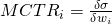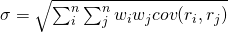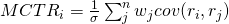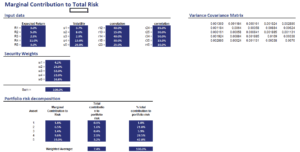# Marginal Contribution To Risk (MCTR)

The Marginal contribution to Risk (MCTR) is a risk measure that is very useful when assessing a portfolio’s riskiness. Essentially the MCTR measures the marginal amount of risk an individual security contributes to overall risk. It can easily be calculated provided we have the covariance matrix and weights of all the securities in the portfolio.

On this page, we discuss the marginal contribution to risk definition and formula and provide a marginal contribution to risk example. It is possible compute the marginal contribution to risk in Excel.

## MCTR definition

What is marginal contribution to risk? MCTR is a very useful measure to determine how much a security is contributing to overall portfolio risk. “Marginal” refers to the incremental risk that is introduced if we would increase the position in a security by a very small amount.

The MCTR of a security to a portfolio can be expressed as followswhere sigma is the volatility of the portfolio and wi is the weight of the security for which we want to calculate the MCTR.

## MCTR formula

First, we need to define the portfolio standard deviationwhere n is the number of assets in the portfolio and cov(ri,rj) is the covariance between security i and security j. Thus, if we have estimates of overall portfolio variance and we have the weights, then we can calculate the MCTR.

The formula for MCTR is the following (basically, we take the first difference of the volatility formula above)It is important to stress that the MCTR does not only depend on the volatility of the security, but also the correlation with the other securities in the portfolio. Also, if the portfolio weight changes considerably, the MCTR itself will change. This is because MCTR is defined for a small change in the weight of the security. For example, suppose we allocate between just two securities. Once one security starts to dominate the portfolio, it’s contribution to risk becomes smaller and smaller. That is because the security is already dominating the portfolio.

## Marginal contribution to active risk

It is also possible to calculate the marginal contribution to active risk. In that case, rather than using the portfolio weights, we use the portfolio active weights to determine active risk.

Marginal contribution to risk Excel

Finally, we implement a very simple example in Excel for five securities to illustrate the calculations. This shows that it is very easy to build a marginal contribution to risk calculator in Excel. The following figure illustrates the calculations. The spreadsheet is available for download at the bottom of the page.Summary

We discussed the marginal contribution to risk, a useful way to analyze the risk contribution of the different securities in the portfolio.

### MCTR Calculator

Want to have an implementation in Excel? Download the Excel file: MCTR